# 重载操作符解析（原）

重载操作符是个好青年，但是要吐槽的是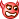，我们时常为了重载操作符编写许多重复的代码。这是枯燥的，但是也是必须的。你重载的越多，你的类的弹性就越大。但是，你也不能为所欲为。玩游戏总是遵守相应的规则，写重载操作符亦是如此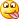以下是要遵守的游戏规则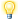1. 一元操作符可以是不带参数的成员函数或带一个参数的非成员函数。
2. 二元操作符可以是带一个参数的成员函数或带两个参数的非成员函数。
3. operator=、operator[]、operator()、operator->只能定义为成员函数。
4. operator->的返回值必须是一个指针或能使用->的对象。
5. 重载 operator++ 和 operator--时带一个 int 参数表示后缀，不带参数表示前缀。
6. 除 operator new 和 operator delete 外，重载的操作符参数中至少要有一个非内建数据类型。
7. 重载的的操作符应尽量模拟操作符对内建类型的行为。

在看完游戏规则之后，我们就各个部分的实现和注意点进行肢解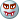⒈ 输入和输出操作符的重载

对于>>和<<的重载要注意以下几个要点① IO操作符必须为非成员函数，如果将其定义为成员函数，那么IO操作符的操作习惯将和正常的习惯相反。多怪啊！此时，你或许会问，那我怎么调用对象中的私有成员呢？别急，我们不是有友员和友类吗？将IO操作符重载函数定义成类的友员函数，这个问题就迎刃而解了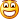② 在输入期间，我们可能会碰到错误。此时，要恢复对象为初始状态。也就是，在输入之前什么样子，我们就恢复成那个样子。

③ 这个要点是摘至《C++ primer》，我觉得挺好的。我们可以重载操作符，意味着我们自由的空间就大了。但是，我们不要忘了IO操作符的本质，不要过度的格式化，应将格式化降到最低。

在注意了几个要点之后，我们看一个完整的IO操作符的实现例子：

#include <iostream>
#include <string>
using namespace std;

class MyClass {
private:
    string name;
    int id;
    int prefix;
    int value;
public:
    MyClass() { };
    MyClass(string n, int a, int p, int nm):name(n), id(a), prefix(p), value(nm){}    // 利用初始化列表来初始化成员对象

    friend ostream &operator<<(ostream &stream, MyClass o);        // 操作符被定义为非成员函数时，要将其定义为所操作类的友员
    friend istream &operator>>(istream &stream, MyClass &o);
};

ostream &operator<<(ostream &stream, MyClass o)
{
    stream << o.name << " ";
    stream << "(" << o.id << ") ";
    stream << o.prefix << "-" << o.value << "\n";

    return stream;
}

istream &operator>>(istream &stream, MyClass &o)
{
    cout << "Enter name: ";
    stream >> o.name;
    cout << "Enter id: ";
    stream >> o.id;
    cout << "Enter prefix: ";
    stream >> o.prefix;
    cout << "Enter value: ";
    stream >> o.value;
    cout << endl;

    return stream;
}

int main()
{
    MyClass a;
    operator>>(cin, a);        // 相当于operator>>(cin, a)
    cout << a;                // 相当于operator<<(cout, a)
    return 0;
}

我觉得，许多的事情都是尽在不言中。看了代码，你就知道，这个家伙是这么用的，这样用才是规范的。好了接下来介绍算术操作符和关系操作符。

void operator<<(ostream &stream, MyClass o)

cout<<obj;

void operator>>(istream &stream, MyClass &o)

cin<<obj;

⒉ 算术操作符和关系操作符的重载

一般而言，将算术操作符和关系操作符定义为非成员函数。

① 算术操作符

那就看代码怎么实现吧：

#include <iostream>
#include <string>

using namespace std;

class Point
{
public:
    Point(){};
    Point(int x_, int y_):x(x_),y(y_){};
    Point(const Point &p){
        this->x = p.x;
        this->y = p.y;
    };

    ~Point(){};

    friend Point operator+(Point &p1, Point &p2);    // 两个对象相加
    friend Point operator+(int value, Point &p1);    // 对象和值的相加

    friend ostream &operator<<(ostream &os, Point &p1);
private:
    int x;
    int y;
};

Point operator+(Point &p1, Point &p2)
{
    Point temp;

    temp.x = p1.x + p2.x;
    temp.y = p1.y + p2.y;

    return temp;
}

Point operator+(int value, Point &p1)
{
    Point temp;

    temp.x = p1.x + value;
    temp.y = p1.y + value;

    return temp;
}

ostream &operator<<(ostream &os, Point &p1)
{
    os << p1.x << " " << p1.y << endl;
    return os;
}

int main()
{
    Point p1(1,2);
    Point p2(3,4);

    cout << p1 + p2;
    cout << 5 + p1;

    return 0;
}

② 相等操作符

首先，“==”相等操作符的两个对象包含相同的数据，这样才有比较性。其次，定义了operator==，同时也要定义operator!=。

friend bool operator==(Point &p1, Point &p2);
friend bool operator!=(Point &p1, Point &p2);

.......

bool operator==(Point &p1, Point &p2)
{
    return (p1.x == p2.x)&&(p1.y == p2.y);
}

bool operator!=(Point &p1, Point &p2)
{
    return !(p1 == p2);
}

⒊ 赋值操作符

赋值操作符有个强调点，那是赋值必须返回对*this的引用。要定义为成员函数。

Point &operator=(const Point &p1);
Point &operator+=(const Point &p1);

.....

Point &Point::operator=(const Point &p1)
{
    this->x = p1.x;
    this->y = p1.y;

    return *this;
}

Point &Point::operator+=(const Point &p1)
{
    this->x += p1.x;
    this->y += p1.y;

    return *this;
}

⒋ 下标操作符

可以从容器中检索单个元素的容器类一般会定义下标操作符operator[]。首先，要注意到，下标操作符必须定义为成员函数。其次，要定义两个版本，一个是非const成员并返回引用。一个是为const成员并返回引用。

#include <iostream>
using namespace std;

class Point {
    int a;
public:
    Point(int i, int j, int k) {
        a = i;
        a = j;
        a = k;
    }
    int &operator[](int &i) { return *(a + i); }
    const int &operator[](const int &i) { return *(a + i); }

};

int main()
{
    Point ob(1, 2, 3);
    cout << ob;
    return 0;
}

在sgi stl中，可以看到重载的情形：（够简洁的reference operator[](size_type __n) { return *(begin() + __n); }
const_reference operator[](size_type __n) const { return *(begin() + __n); }

⒌ 成员访问操作符

C++中支持重载解引用操作符（*）和箭头操作符（->），其中，箭头操作符必须定义为类成员函数，解引用则两者皆可。看看以下的用法：

_Reference operator*() const {
  _BidirectionalIterator __tmp = current;
  return *--__tmp;    // 返回值
}

pointer operator->() const { return &(operator*()); }   // 返回指针

⒍ 自增和自减操作

a++，++a，--b，b--。是不是有点烦人？但是看了重载的意义之后，你就知道，这个东西是不烦人的。也知道了在for循环中为什么要强调用++a了。

在C++中，并没有特别要求说一定要为成员函数，但是为成员函数是一个不错的选择还有要注意的是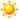① 为了与内置类型一致，前缀式操作符应返回被增量或减量对象的引用；

② 后缀式返回旧值，应作为值返回，不是返回引用，所以返回不用引用。

现在看看如何使用:

Point &operator++();    // 为了与内置类型一致，前缀式操作符应返回被增量或减量对象的引用
Point operator++(int);    // 返回旧值，应作为值返回，不是返回引用

Point &operator--();
Point operator--(int);
....
// 前增
Point &Point::operator++()
{
    ++this->x;
    ++this->y;
    return *this;
}

// 后增
Point Point::operator++(int)
{
    Point temp = *this;
    ++this->x;
    ++this->y;
    return temp;
}

// 前减
Point &Point::operator--()
{
    --this->x;
    --this->y;
    return *this;
}

// 后减
Point Point::operator--(int)
{
    Point temp = *this;
    --this->x;
    --this->y;
    return temp;
}

知道为什么说要强调说前缀式了吗？看看前缀和后缀的区别，你就知道那个效率会高。。。

总结，这些东西写的有点辛苦，写写停停的。请大牛指正其中不妥之处，小菜谢谢了。。。

05-1494
02-2139012-23798
02-21571
08-29158
09-07104
04-04304